# ThinkDSP¶

by Allen Downey (think-dsp.com)

This notebook contains examples and demos for a SciPy 2015 talk.

In :
import thinkdsp
from thinkdsp import decorate

import numpy as np


A Signal represents a function that can be evaluated at an point in time.

In :
cos_sig = thinkdsp.CosSignal(freq=440)


A cosine signal at 440 Hz has a period of 2.3 ms.

In :
cos_sig.plot()
decorate(xlabel='time (s)')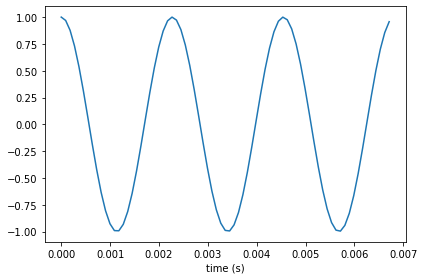make_wave samples the signal at equally-space time steps.

In :
wave = cos_sig.make_wave(duration=0.5, framerate=11025)


make_audio creates a widget that plays the Wave.

In :
wave.apodize()
wave.make_audio()

Out:

make_spectrum returns a Spectrum object.

In :
spectrum = wave.make_spectrum()


A cosine wave contains only one frequency component (no harmonics).

In :
spectrum.plot()
decorate(xlabel='frequency (Hz)')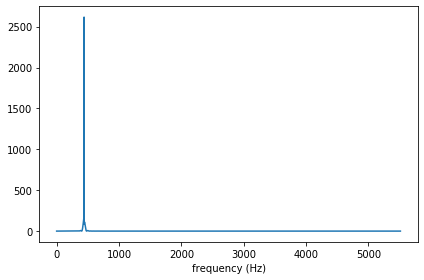A SawTooth signal has a more complex harmonic structure.

In :
saw_sig = thinkdsp.SawtoothSignal(freq=440)
saw_sig.plot()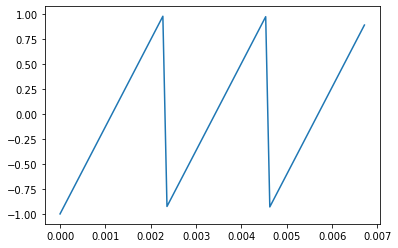Here's what it sounds like:

In :
saw_wave = saw_sig.make_wave(duration=0.5)
saw_wave.make_audio()

Out:

And here's what the spectrum looks like:

In :
saw_wave.make_spectrum().plot()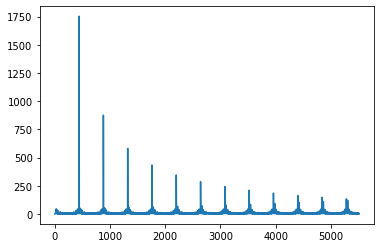Here's a short violin performance from jcveliz on freesound.org:

In :
violin = thinkdsp.read_wave('92002__jcveliz__violin-origional.wav')
violin.make_audio()

Out:

The spectrogram shows the spectrum over time:

In :
spectrogram = violin.make_spectrogram(seg_length=1024)
spectrogram.plot(high=5000)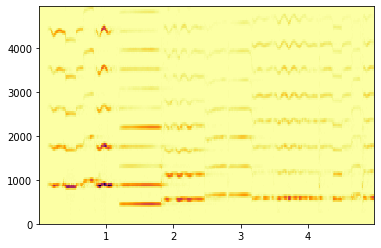We can select a segment where the pitch is constant:

In :
start = 1.2
duration = 0.6
segment = violin.segment(start, duration)


And compute the spectrum of the segment:

In :
spectrum = segment.make_spectrum()
spectrum.plot()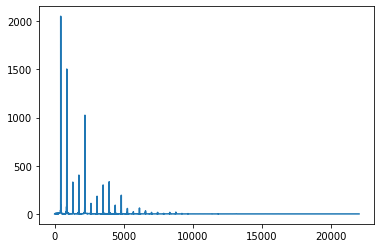The dominant and fundamental peak is at 438.3 Hz, which is a slightly flat A4 (about 7 cents).

In :
spectrum.peaks()[:5]

Out:
[(2052.3878454763044, 438.33333333333337),
(1504.1231272792363, 876.6666666666667),
(1313.4058092162186, 878.3333333333334),
(1024.7130064064418, 2193.3333333333335),
(809.7623839848649, 2195.0)]

As an aside, you can use the spectrogram to help extract the Parson's code and then identify the song.

Parson's code: DUUDDUURDR

Send it off to http://www.musipedia.org

A chirp is a signal whose frequency varies continuously over time (like a trombone).

In :
import math
PI2 = 2 * math.pi

class SawtoothChirp(thinkdsp.Chirp):
"""Represents a sawtooth signal with varying frequency."""

def _evaluate(self, ts, freqs):
"""Helper function that evaluates the signal.

ts: float array of times
freqs: float array of frequencies during each interval
"""
dts = np.diff(ts)
dps = PI2 * freqs * dts
phases = np.cumsum(dps)
phases = np.insert(phases, 0, 0)
cycles = phases / PI2
frac, _ = np.modf(cycles)
ys = thinkdsp.normalize(thinkdsp.unbias(frac), self.amp)
return ys


Here's what it looks like:

In :
signal = SawtoothChirp(start=220, end=880)
wave = signal.make_wave(duration=2, framerate=10000)
segment = wave.segment(duration=0.06)
segment.plot()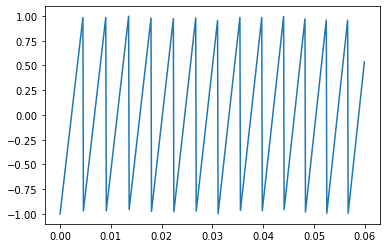Here's the spectrogram.

In :
spectrogram = wave.make_spectrogram(1024)
spectrogram.plot()
decorate(xlabel='Time (s)', ylabel='Frequency (Hz)')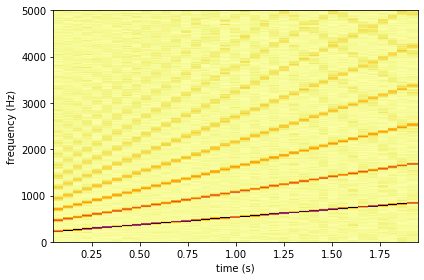What do you think it sounds like?

In :
wave.apodize()
wave.make_audio()

Out:

Up next is one of the coolest examples in Think DSP. It uses LTI system theory to characterize the acoustics of a recording space and simulate the effect this space would have on the sound of a violin performance.

In :
response = thinkdsp.read_wave('180960__kleeb__gunshot.wav')

start = 0.12
response = response.segment(start=start)
response.shift(-start)

response.normalize()
response.plot()
decorate(xlabel='Time (s)', ylabel='amplitude')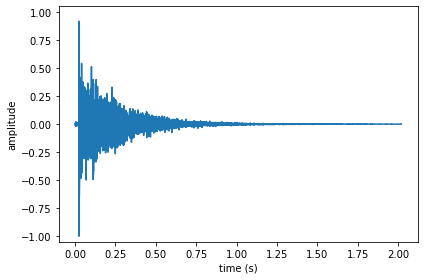If you play this recording, you can hear the initial shot and several seconds of echos.

In :
response.make_audio()

Out: